/

### Performance Measurement Theory 2. Mathematics of multi-period analysis

Next page

2.1          Suppose that we are interested in calculating the rate of return on a portfolio from time 0 to time 1 using some suitable units of time. Suppose that there are n new money payments into or out of the portfolio in the period of value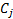(positive for inflows, negative for outflows) occurring at times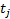for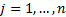. Theare assumed to be ordered so that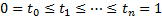. The market values at the corresponding points in time (immediately after receipt of the new money) are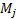. Dividend/interest payments are treated as outflows from the relevant stock/bond sector and inflows into the cash sector, and so net to zero at the total fund level (unless the income is paid away).

2.2          The time-weighted rate of return for the period is then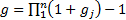where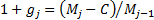. The time-weighted rate of return is effectively equivalent to the growth in a unit net asset value price (were the fund to be unitised and were it to accumulate income internally, ignoring complications such as bid/offer spreads, etc.) The positive or negative impact of money arriving or being withdrawn from the portfolio at opportune or inopportune times is stripped out of the calculation. Time-weighted rates of return naturally compound up over time, i.e. if the time-weighted rate of return in one period is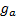and in the next is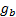then the time weighted rate of return for the combined period is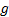where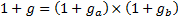. This also means that ‘logged’ returns, i.e.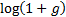, naturally add up through time, i.e.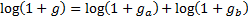.

2.3          The money-weighted or internal rate of return on a fund over the same period, is defined as the ‘sensible’ solution forto the following equation (if theare of differing signs then there will usually be more than one solution, although normally only one would be intrinsically ‘sensible’):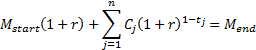2.4          The time-weighted rate of return and the money-weighted rate of return are thus the same if there have been no cash flows during the period.

2.5          One nearly always assumes that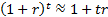. The internal rate of return can therefore be approximated by the formula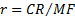, where the contribution to return (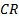), net new money (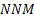), time-weighted net investment (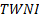), and mean fund (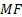), are defined as follows: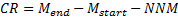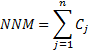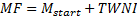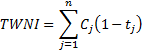2.6          The internal rate of return is the (constant) interest rate that a bank account would need to provide (possibly negative) to return the same amount at the end of the period as the portfolio, given the same new money flows and the same start market value. It is therefore the same as the money-weighted rate of return and so again does not naturally compound up over time.

2.7          Calculating the time-weighted rate of return in principle involves valuations whenever there is a cash flow. This can be time consuming, unless you have an exceptionally good valuation engine (and even then is potentially impossible if you wish to value at the exact intra-day point of time at which a particular trade takes place).

2.8          In practice, therefore, performance measurers often merely chain-link internal rates of return. This is because the money weighted and time weighted rates of return are the same if there are no intra-period new money flows. So, if you calculate internal rates of return sufficiently often and chain-link them together then the result will always tend to the time-weighted rate of return.

In certain other special circumstances, the money-weighted and time-weighted rates of return are also identical. Normally, cash flows and market values will be expressed in some base currency, but suppose we generalise the calculation of money-weighted rates of return so that it can include an arbitrary calculation numeraire, which is worth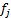in the base currency at timeThe money-weighted rate of return then becomeswhere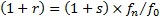and whereis the solution to: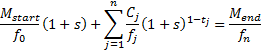2.9          The money-weighted rate of return described above is then merely a special case of this calculation with a constant (in base currency) numeraire. Suppose that we choose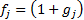, where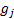corresponds to the true cumulative time-weighted return from time 0 to timeThen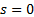and the money-weighted rate of return,, will (in this numeraire) be identical to the time-weighted rate of return. Ifclosely approximates to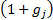thenwill closely approximate to 0, and the approximation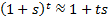will be very good. The money-weighted rate of return using such a numeraire will then be very similar to the true time-weighted rate of return. If the new money flows are small in relation to start and end market values then the money-weighted rate of return will also be very similar to the true time-weighted rate of return, irrespective of the calculation numeraire.

2.10        The calculation numeraire can be differentiated from the presentation numeraire used to express the results of the calculation, which will normally be the base currency of the portfolio. If the presentation numeraire is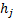then the rates of return would be restated to be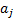where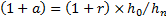.

2.11        The above approach requires not only fund holding and valuation price data but also information on the prices at which individual transactions were carried out. If these are difficult to obtain then an alternative, less exact, methodology involves buy and hold attribution. In this methodology, the return on each line of stock is imputed merely from market data over a given period (usually daily) on the assumption that no transactions have taken place. Such an approach produces the same answer as a true transactions-based analysis either if no transactions occur or if they occur at the prices assumed in the algorithm. Unfortunately this approximation can lead to significant residuals for funds with high turnover or subject to significant dealing costs.